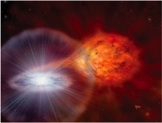Search:# Observation Analysis

Here we describe the analysis procedures for the observations incorporated into the sample.

## BeppoSAX Wide Field Camera

The BeppoSAX Wide Field Cameras (WFCs) were operational between June 24, 1996, and April 30, 2002. The two identical cameras pointed in opposite directions with fields of views of $40\times40$ square degrees, encompassing 4% of the sky each. The total useable exposure time over the whole mission is a strong function of sky position, but is mostly between 1 and 12 Msec.

The sensitivity is a strong function of the source position within the field of view and the total flux from all sources contained in the field of view. For the on-axis position, the sensitivity on a time scale of 1 s is at best 0.2 Crab units and at worst 0.8 Crab units above the background (at a 5$\sigma$ threshold and for 3-25 keV).

Observations, with a fixed pointing, lasted between $10^3$ s and 9 days. Most observations lasted about 1 d.

• effective area; spectral resolution; references
• persistent spectral models and fit approach
• lightcurve generation
• define Crab units

Jean in 't Zand 30.4.2011

## RXTE Proportional Counter Array

We estimated the persistent source flux $F_p$ for each RXTE observation from spectra extracted from Standard-2 mode data, separately for each PCU (excluding an interval beginning 32~s before and ending 256~s after each burst). We estimated the deadtime correction using the Standard-1 mode data\footnote{following the recipe at {\url http://heasarc.gsfc.nasa.gov/docs/xte/recipes/pca_deadtime.html}} and applied the correction by reducing the exposure time by the calculated deadtime correction factor. The correction factor was in the range ... We estimated the instrumental background for each PCU over each interval in which it was active using the all-mission background model file appropriate for bright'' sources ($\gtrsim40$~counts~s$^{-1}$~PCU$^{-1}$).

comment here on the effect of using the bright source background where it is not appropriate

For PCU\#1, we found that the background estimation was a poor match to the on-source spectrum from 2010 October through to the mission end. For sources with PCU\#1 active in this time interval, we neglected the spectra from PCU\#1 in the fits. to be reviewed

We fit the net spectra over the range 2.5-25 keV with an empirical model consisting of a continuum component, either a power law; blackbody and power-law ({\tt bbodyrad+powerlaw} in {\sc xspec}); or a comptonisation spectrum ({\tt comptt}), each attenuated by neutral absorption with solar abundances. model choice to be revised

For many of the spectra, residuals were present around 6.4 keV which we interpreted as fluorescent Fe K$\alpha$ emission, and where these residuals resulted in a reduced $\chi^2\gtrsim 2$ we added a Gaussian component to improve the fit. For particularly bright sources, such a model did not give a good fit, and for these we used instead a continuum component describing Comptonization in a homogeneous environment ({\tt compTT} in {\sc xspec}; Titarchuk 1994b). The particular choice of the continuum did not significantly affect the measured flux within the energy range covered by the PCA.

• describe criterion for model selection
• add statistics on various models

We fixed the neutral absorption column density $n_H$ to the tabled value for each source in all the fits, for consistency.

We then integrated the model over the energy range 2.5--25~keV\footnote{The Crab flux in this band is $3.3\times10^{-8}\ {\rm erg\,cm^{-2}\,s^{-1}$} to estimate the source flux detected by each PCU.

For each source we calculated the mean PCU-to-PCU offset averaged over all the public RXTE observations, and renormalized the flux measurements relative to PCU \#2. We adopted the mean and standard deviation of the rescaled flux measurements as the flux and error for that observation. to be done

While the majority of the burst flux is emitted in the range 2.5--25~keV, this is generally not true for the persistent emission. In order to estimate the bolometric persistent flux $F_{\rm bol}$, we chose representative (preferably long) observations for selected sources and undertook combined fits of each PCU spectra (as described above) along with HEXTE spectra above 15~keV. We set the upper energy limit for the HEXTE spectra individually depending on the maximum energy to which the source could be detected (typically 40--80~keV ). Persistent spectra from bursters frequently exhibit a spectral cutoff between 15 and 50 keV, and so we fit the broadband spectra with a Comptonization continuum component attenuated by neutral absorption, also sometimes with a Gaussian component representing fluorescent Fe K$\alpha$ emission around 6.4 keV. We generated an idealized response covering the energy range 0.1--200~keV with 200 logarithmically spaced energy bins, and also integrated the model flux over this range. We then calculated a bolometric correction $c_{\rm bol}$ as the ratio between the 0.1--200 and 2.5--25~keV fluxes measured from the broadband spectral fits. The error on the bolometric correction was estimated as the standard deviation of the derived correction over the available PCUs. Altogether we estimated bolometric corrections for observations of 24 bursting sources, ranging between 1.05 (from a 1997 September observation of 4U~1728$-$34) and 2.14 (for a 2002 October observation of SAX~J1808.4$-$3658; Table 2). The corrections for the accretion- powered pulsars tended to be larger than for the nonpulsing burst sources, and we found the maximum value for the latter sources to be 1.93. In the mean, $c_{\rm bol} = 1.38$ for the nonpulsing sources, and we adopt this value except where we calculated a correction specifically for that source or observation. The likely error introduced is thus no more than $\approx40$\%. to be updated